Next: Estimating camera response function Up: .  Introduction: Variable gain image Previous: Quantimetric imaging

## Estimation in the presence of noise

Consider first, a special case in which we know each of the relative exposure values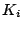. For this special case, images are related to to the actual quantity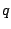as well as to each other, by:

f_i (x) = f( k_i q ( x ) +n_q_i ) +n_f_i where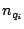includes sensor noise, and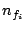includes image noise due to quantization, compression, transmission. (For precise definitions of these two noise sources, see .)

In the presence of noise, each picture provides an estimate of the actual quantity of light falling on the image sensor: q_i (x) = 1k_i f^-1(f_i( x)) where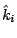is an estimate of the actual exposure constant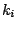, andis an estimate of the true camera response function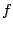, assuming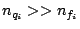.

Multiple estimates of the actual quantity of light falling on the image sensor may be combined as follows: q(x) = _i c_i q_i(x) _i c_i

Photographic film is traditionally characterized by the so-called Density versus log Exposure'' characteristic curvewyckoff. Similarly, in the case of electronic imaging, we may also use logarithmic exposure units,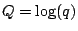, so that one image will be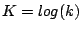units darker than the other: (f^-1(f_1(x))) =Q =( f^-1( f_2( x ) ) ) - K The existence of an inverse forfollows from a semimonotonicity assumption. Semimonotonicity follows from the fact that we expect pixel values to either increase or stay the same with increasing quantity of illumination,1. Since the logarithm function is also monotonic, the problem comes down to estimating the semimonotonic function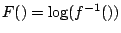and the scalar constant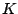.

The unknowns (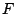and) may be solved in a least squares sense2.Next: Estimating camera response function Up: .  Introduction: Variable gain image Previous: Quantimetric imaging
Steve Mann 2002-05-25